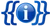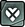Documentation

This the Infobox for Heroes.

## Usage

```{{Infobox hero
|name           =
|alliance1      =
|alliance2      =
|alliance3      =
|tier           =
|health1        =
|health2        =
|health3        =
|mana1          =
|mana2          =
|mana3          =
|damage1        =
|damage2        =
|damage3        =
|attackspeed1   =
|attackspeed2   =
|attackspeed3   =
|movespeed1     =
|movespeed2     =
|movespeed3     =
|attackrange1   =
|attackrange2   =
|attackrange3   =
|magicresist1   =
|magicresist2   =
|magicresist3   =
|armor1         =
|armor2         =
|armor3         =
|healthregen1   =
|healthregen2   =
|healthregen3   =
}}
```

## ExampleTier Three
Level750 1,500 3,000
100 100 100
38 77 154
[45/55] [90/110] [180/220]
1.30 1.30 1.30
Move Speed
325 325 325
1 1 1
0 0 0
10 10 10
```{{Infobox hero
|alliance1      = Heartless
|alliance2      = Knight
|alliance3      =
|tier           = 3
|health1        = 750
|health2        = 1500
|health3        = 3000
|mana1          = 100
|mana2          = 100
|mana3          = 100
|damage1        = [45/55]
|damage2        = [90/110]
|damage3        = [180/220]
|attackspeed1   = 1.30
|attackspeed2   = 1.30
|attackspeed3   = 1.30
|movespeed1     = 325
|movespeed2     = 325
|movespeed3     = 325
|attackrange1   = 1
|attackrange2   = 1
|attackrange3   = 1
|magicresist1   = 0
|magicresist2   = 0
|magicresist3   = 0
|armor1         = 10
|armor2         = 10
|armor3         = 10
|healthregen1   = 0
|healthregen2   = 0
|healthregen3   = 0
}}
```